Download Presentation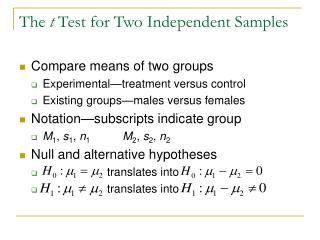The t Test for Two Independent Samples

# The t Test for Two Independent Samples - PowerPoint PPT Presentation

The t Test for Two Independent Samples Compare means of two groups Experimental—treatment versus control Existing groups—males versus females Notation—subscripts indicate group M 1 , s 1 , n 1 M 2 , s 2 , n 2 Null and alternative hypothesesI am the owner, or an agent authorized to act on behalf of the owner, of the copyrighted work described.
Download Presentation## The t Test for Two Independent Samples

An Image/Link below is provided (as is) to download presentation

Download Policy: Content on the Website is provided to you AS IS for your information and personal use and may not be sold / licensed / shared on other websites without getting consent from its author.While downloading, if for some reason you are not able to download a presentation, the publisher may have deleted the file from their server.

- - - - - - - - - - - - - - - - - - - - - - - - - - E N D - - - - - - - - - - - - - - - - - - - - - - - - - -
Presentation Transcript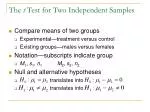The t Test for Two Independent Samples
• Compare means of two groups
• Experimental—treatment versus control
• Existing groups—males versus females
• Notation—subscripts indicate group
• M1, s1, n1 M2, s2, n2
• Null and alternative hypotheses
• translates into
• translates into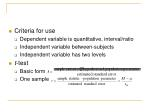Criteria for use
• Dependent variable is quantitative, interval/ratio
• Independent variable between-subjects
• Independent variable has two levels
• t-test
• Basic form
• One sample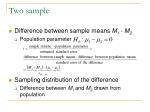Two sample
• Difference between sample means M1 - M2
• Population parameter
• Sampling distribution of the difference
• Difference between M1 and M2 drawn from population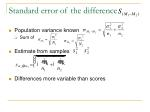Standard error of the difference
• Population variance known
• Sum of
• Estimate from samples
• Differences more variable than scores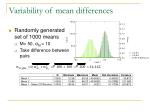Variability of mean differences
• Randomly generated set of 1000 means
• Μ= 50, σM = 10
• Take difference between pairs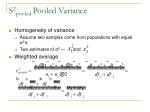S2pooled Pooled Variance
• Homogeneity of variance
• Assume two samples come from populations with equal σ2’s
• Two estimates of σ2 — and
• Weighted average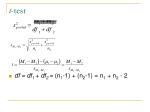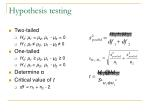Hypothesis testing
• Two-tailed
• H0: µ1 = µ2, µ1 - µ2 = 0
• H1: µ1 ≠ µ2, µ1 - µ2 ≠ 0
• One-tailed
• H0: µ1 ≥ µ2, µ1 - µ2 ≥ 0
• H1: µ1 < µ2, µ1 - µ2 < 0
• Determine α
• Critical value of t
• df = n1 + n2 - 2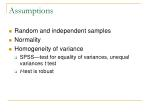Assumptions
• Random and independent samples
• Normality
• Homogeneity of variance
• SPSS—test for equality of variances, unequal variances t test
• t-test is robust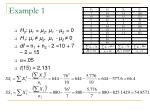H0: µ1 = µ2, µ1 - µ2 = 0

H1: µ1 ≠ µ2, µ1 - µ2 ≠ 0

df = n1 + n2 - 2 =10 + 7 – 2 = 15

=.05

t(15) = 2.131

Example 1Confidence Interval for the Difference
• Example 1
• -3.257 - (2.131*1.401) < µ1 - µ2 < -3.257 + (2.131*1.401) = -6.243 < µ1 - µ2 < -0.272
• Example 2
• -0.867 - (1.701*5.221) < µ1 - µ2 < -0.867 + (1.701*5.221) = -9.748 < µ1 - µ2 < 8.014
• Includes 0 retain H0SPSS
• Analyze
• Compare Means
• Independent-Samples T Test
• Dependent variable(s)—Test Variable(s)
• Independent variable—Grouping Variable
• Define Groups
• Cut point value
• Output
• Levene’s Test for Equality of Variances
• t Tests
• Equal variances assumed
• Equal variances not assumedEffect size
• Cohen’s d =
• Example 1 Cohen’s d
• Example 2 Cohen’s d
• r2 or η2
• G = grand meanFactors Influencing t–test and Effect Size
• Mean difference M1 – M2
• Larger difference, larger t
• Larger difference, larger r2 and Cohen’s d• M1 – M2 increases from –3.257 to –6.257
• unaffected t increases from –2.325 to –4.466
• r2increases fromMagnitude of sample variances
• As sample variances increase:
• t decreases
• Cohen’s d and r2 decreases
• SSExplainedunchanged, SSErrorand SSTotal increases, S2pooled increasesSample size
• Larger sample smaller t affects
• No effect on Cohen’s d, minimal effect on r2
• First example increase n1from 10 to 30 and n2 from 7 to 21